# NCERT Exemplar solutions for Class 11 Physics chapter 3 - Motion In a Straight Line [Latest edition]

#### Chapters## Solutions for Chapter 3: Motion In a Straight Line

Below listed, you can find solutions for Chapter 3 of CBSE NCERT Exemplar for Class 11 Physics.

Exercises
Exercises [Pages 13 - 18]

### NCERT Exemplar solutions for Class 11 Physics Chapter 3 Motion In a Straight Line Exercises [Pages 13 - 18]

#### MCQ I

Exercises | Q 3.1 | Page 13

Among the four graphs (Figure), there is only one graph for which average velocity over the time intervel (0, T ) can vanish for a suitably chosen T. Which one is it?

•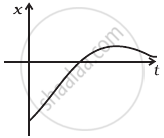•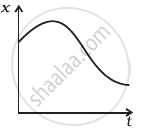••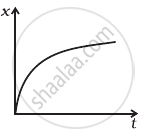Exercises | Q 3.2 | Page 14

A lift is coming from 8th floor and is just about to reach 4th floor. Taking ground floor as origin and positive direction upwards for all quantities, which one of the following is correct?

• x < 0, v < 0, a > 0

• x > 0, v < 0, a < 0

• x > 0, v < 0, a > 0

• x > 0, v > 0, a < 0

Exercises | Q 3.3 | Page 14

In one dimensional motion, instantaneous speed v satisfies 0 ≤ v < v0.

• The displacement in time T must always take non-negative values.

• The displacement x in time T satisfies – v0T < x < v0T.

• The acceleration is always a non-negative number.

• The motion has no turning points.

Exercises | Q 3.4 | Page 14

A vehicle travels half the distance L with speed V1 and the other half with speed V2, then its average speed is ______.

• (V_1 + V_2)/2

• (2V_1 + V_2)/(V_1 + V_2)

• (2V_1 V_2)/(V_1 + V_2)

• (L(V_1 + V_2))/(V_1V_2)

Exercises | Q 3.5 | Page 14

The displacement of a particle is given by x = (t – 2)2 where x is in metres and t in seconds. The distance covered by the particle in first 4 seconds is ______.

• 4 m

• 8 m

• 12 m

• 16 m

Exercises | Q 3.6 | Page 14

At a metro station, a girl walks up a stationary escalator in time t1. If she remains stationary on the escalator, then the escalator take her up in time t2. The time taken by her to walk up on the moving escalator will be ______.

• ((t_1 + t_2))/2

• ((t_1t_2))/((t_2 - t_1))

• ((t_1t_2))/((t_2 + t_1))

• (t_1 - t_2)

#### MCQ II

Exercises | Q 3.7 | Page 15

The variation of quantity A with quantity B, plotted in figure describes the motion of a particle in a straight line.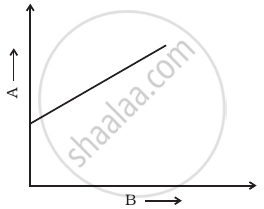1. Quantity B may represent time.
2. Quantity A is velocity if motion is uniform.
3. Quantity A is displacement if motion is uniform.
4. Quantity A is velocity if motion is uniformly accelerated.
Exercises | Q 3.8 | Page 15

A graph of x versus t is shown in figure. Choose correct alternatives from below.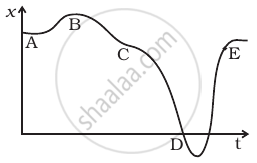1. The particle was released from rest at t = 0.
2. At B, the acceleration a > 0.
3. At C, the velocity and the acceleration vanish.
4. Average velocity for the motion between A and D is positive.
5. The speed at D exceeds that at E.
Exercises | Q 3.9 | Page 15

For the one-dimensional motion, described by x = t – sint

1. x (t) > 0 for all t > 0.
2. v (t) > 0 for all t > 0.
3. a (t) > 0 for all t > 0.
4. v (t) lies between 0 and 2.
Exercises | Q 3.10 | Page 15

A spring with one end attached to a mass and the other to a rigid support is stretched and released.

1. Magnitude of acceleration, when just released is maximum.
2. Magnitude of acceleration, when at equilibrium position, is maximum.
3. Speed is maximum when mass is at equilibrium position.
4. Magnitude of displacement is always maximum whenever speed is minimum.
Exercises | Q 3.11 | Page 16

A ball is bouncing elastically with a speed 1 m/s between walls of a railway compartment of size 10 m in a direction perpendicular to walls. The train is moving at a constant velocity of 10 m/s parallel to the direction of motion of the ball. As seen from the ground ______.

1. the direction of motion of the ball changes every 10 seconds.
2. speed of ball changes every 10 seconds.
3. average speed of ball over any 20 second interval is fixed.
4. the acceleration of ball is the same as from the train.

#### VSA

Exercises | Q 3.12 | Page 16

Refer to the graphs in figure. Match the following.

 Graph Characteristic (a)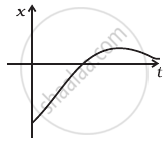(i) has v > 0 and a < 0 throughout. (b)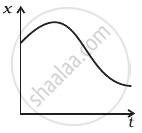(ii) has x > 0 throughout and has a point with v = 0 and a point with a = 0. (c)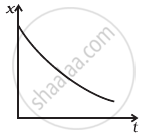(iii) has a point with zero displacement for t > 0. (d)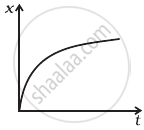(iv) has v < 0 and a > 0.
Exercises | Q 3.13 | Page 16

A uniformly moving cricket ball is turned back by hitting it with a bat for a very short time interval. Show the variation of its acceleration with time. (Take acceleration in the backward direction as positive).

Exercises | Q 3.14 | Page 16

Give examples of a one-dimensional motion where

1. the particle moving along positive x-direction comes to rest periodically and moves forward.
2. the particle moving along positive x-direction comes to rest periodically and moves backward.
Exercises | Q 3.15 | Page 16

Give example of a motion where x > 0, v < 0, a > 0 at a particular instant.

Exercises | Q 3.16 | Page 16

An object falling through a fluid is observed to have acceleration given by a = g – bv where g = gravitational acceleration and b is constant. After a long time of release, it is observed to fall with constant speed. What must be the value of constant speed?

#### SA

Exercises | Q 3.17 | Page 17

A ball is dropped and its displacement vs time graph is as shown figure (displacement x is from ground and all quantities are +ve upwards).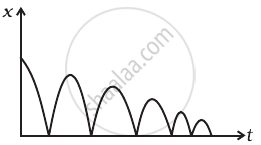1. Plot qualitatively velocity vs time graph.
2. Plot qualitatively acceleration vs time graph.
Exercises | Q 3.18 (a) | Page 17

A particle executes the motion described by x(t) = x0 (1 − e − γt); t ≥ 0, x0 > 0. Where does the particle start and with what velocity?

Exercises | Q 3.18 (b) | Page 17

A particle executes the motion described by x(t) = x0(1 − e −γt); t ≥ 0, x0 > 0. Find maximum and minimum values of x(t), v(t), a(t). Show that x(t) and a(t) increase with time and v(t) decreases with time.

Exercises | Q 3.19 | Page 17

A bird is tossing (flying to and fro) between two cars moving towards each other on a straight road. One car has a speed of 18 m/h while the other has the speed of 27km/h. The bird starts moving from first car towards the other and is moving with the speed of 36km/h and when the two cars were separted by 36 km. What is the total distance covered by the bird? What is the total displacement of the bird?

Exercises | Q 3.20 | Page 17

A man runs across the roof-top of a tall building and jumps horizontally with the hope of landing on the roof of the next building which is of a lower height than the first. If his speed is 9 m/s, the (horizontal) distance between the two buildings is 10 m and the height difference is 9 m, will he be able to land on the next building? (take g = 10 m/s2)

Exercises | Q 3.21 | Page 17

A ball is dropped from a building of height 45 m. Simultaneously another ball is thrown up with a speed 40 m/s. Calculate the relative speed of the balls as a function of time.

Exercises | Q 3.22 | Page 17

The velocity-displacement graph of a particle is shown in figure.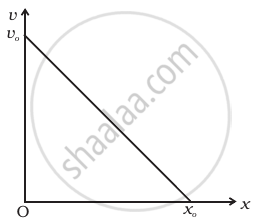1. Write the relation between v and x.
2. Obtain the relation between acceleration and displacement and plot it.

#### LA

Exercises | Q 3.23 | Page 18

It is a common observation that rain clouds can be at about a kilometre altitude above the ground.

1. If a rain drop falls from such a height freely under gravity, what will be its speed? Also calculate in km/h. ( g = 10 m/s2)
2. A typical rain drop is about 4mm diameter. Momentum is mass x speed in magnitude. Estimate its momentum when it hits ground.
3. Estimate the time required to flatten the drop.
4. Rate of change of momentum is force. Estimate how much force such a drop would exert on you.
5. Estimate the order of magnitude force on umbrella. Typical lateral separation between two rain drops is 5 cm.

(Assume that umbrella is circular and has a diameter of 1 m and cloth is not pierced through !!)

Exercises | Q 3.24 | Page 18

A motor car moving at a speed of 72 km/h can not come to a stop in less than 3.0 s while for a truck this time interval is 5.0 s. On a highway the car is behind the truck both moving at 72 km/h. The truck gives a signal that it is going to stop at emergency. At what distance the car should be from the truck so that it does not bump into (collide with) the truck. Human response time is 0.5 s.

Exercises | Q 3.25 | Page 18

A monkey climbs up a slippery pole for 3 seconds and subsequently slips for 3 seconds. Its velocity at time t is given by v(t) = 2t (3 – t); 0 < t < 3 and v(t) = – (t – 3)(6 – t) for 3 < t < 6 s in m/s. It repeats this cycle till it reaches the height of 20 m.

1. At what time is its velocity maximum?
2. At what time is its average velocity maximum?
3. At what time is its acceleration maximum in magnitude?
4. How many cycles (counting fractions) are required to reach the top?
Exercises | Q 3.26 | Page 18

A man is standing on top of a building 100 m high. He throws two balls vertically, one at t = 0 and other after a time interval (less than 2 seconds). The later ball is thrown at a velocity of half the first. The vertical gap between first and second ball is +15 m at t = 2 s. The gap is found to remain constant. Calculate the velocity with which the balls were thrown and the exact time interval between their throw.

## Solutions for Chapter 3: Motion In a Straight Line

Exercises## NCERT Exemplar solutions for Class 11 Physics chapter 3 - Motion In a Straight Line

Shaalaa.com has the CBSE Mathematics Class 11 Physics CBSE solutions in a manner that help students grasp basic concepts better and faster. The detailed, step-by-step solutions will help you understand the concepts better and clarify any confusion. NCERT Exemplar solutions for Mathematics Class 11 Physics CBSE 3 (Motion In a Straight Line) include all questions with answers and detailed explanations. This will clear students' doubts about questions and improve their application skills while preparing for board exams.

Further, we at Shaalaa.com provide such solutions so students can prepare for written exams. NCERT Exemplar textbook solutions can be a core help for self-study and provide excellent self-help guidance for students.

Concepts covered in Class 11 Physics chapter 3 Motion In a Straight Line are Acceleration (Average and Instantaneous), Relative Velocity, Kinematic Equations for Uniformly Accelerated Motion, Elementary Concept of Differentiation and Integration for Describing Motion, Uniform and Non-uniform Motion, Position, Path Length and Displacement, Average Velocity and Average Speed, Instantaneous Velocity and Speed, Uniformly Accelerated Motion, Position-time, Velocity-time and Acceleration-time Graphs, Position - Time Graph, Relations for Uniformly Accelerated Motion (Graphical Treatment), Introduction of Motion in One Dimension.

Using NCERT Exemplar Class 11 Physics solutions Motion In a Straight Line exercise by students is an easy way to prepare for the exams, as they involve solutions arranged chapter-wise and also page-wise. The questions involved in NCERT Exemplar Solutions are essential questions that can be asked in the final exam. Maximum CBSE Class 11 Physics students prefer NCERT Exemplar Textbook Solutions to score more in exams.

Get the free view of Chapter 3, Motion In a Straight Line Class 11 Physics additional questions for Mathematics Class 11 Physics CBSE, and you can use Shaalaa.com to keep it handy for your exam preparation.